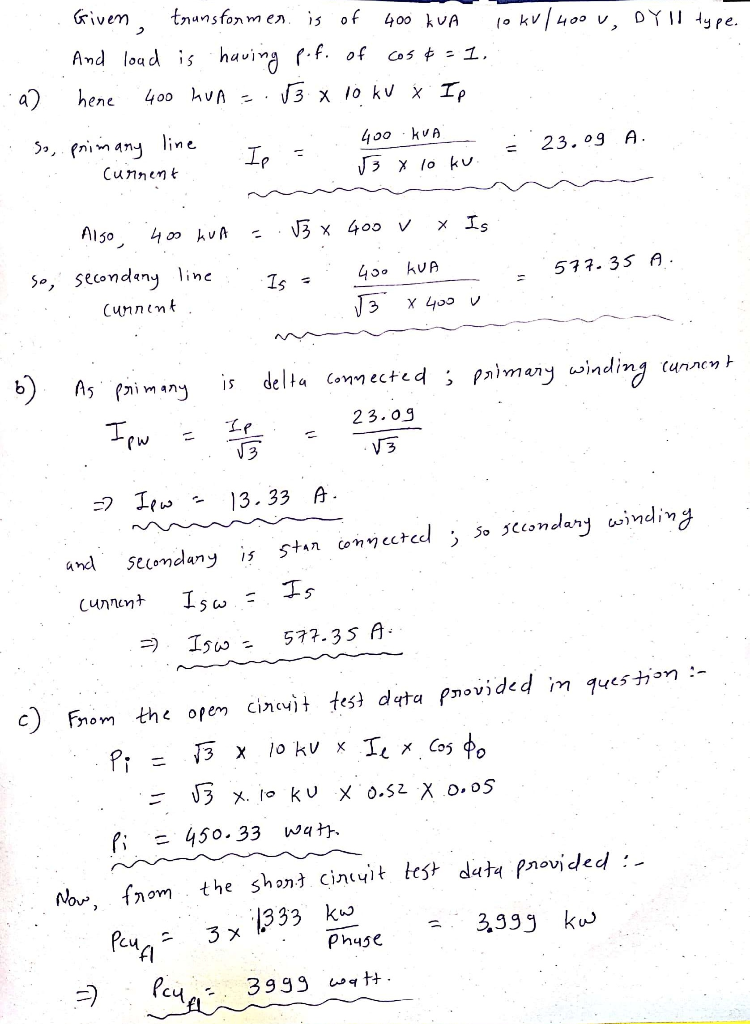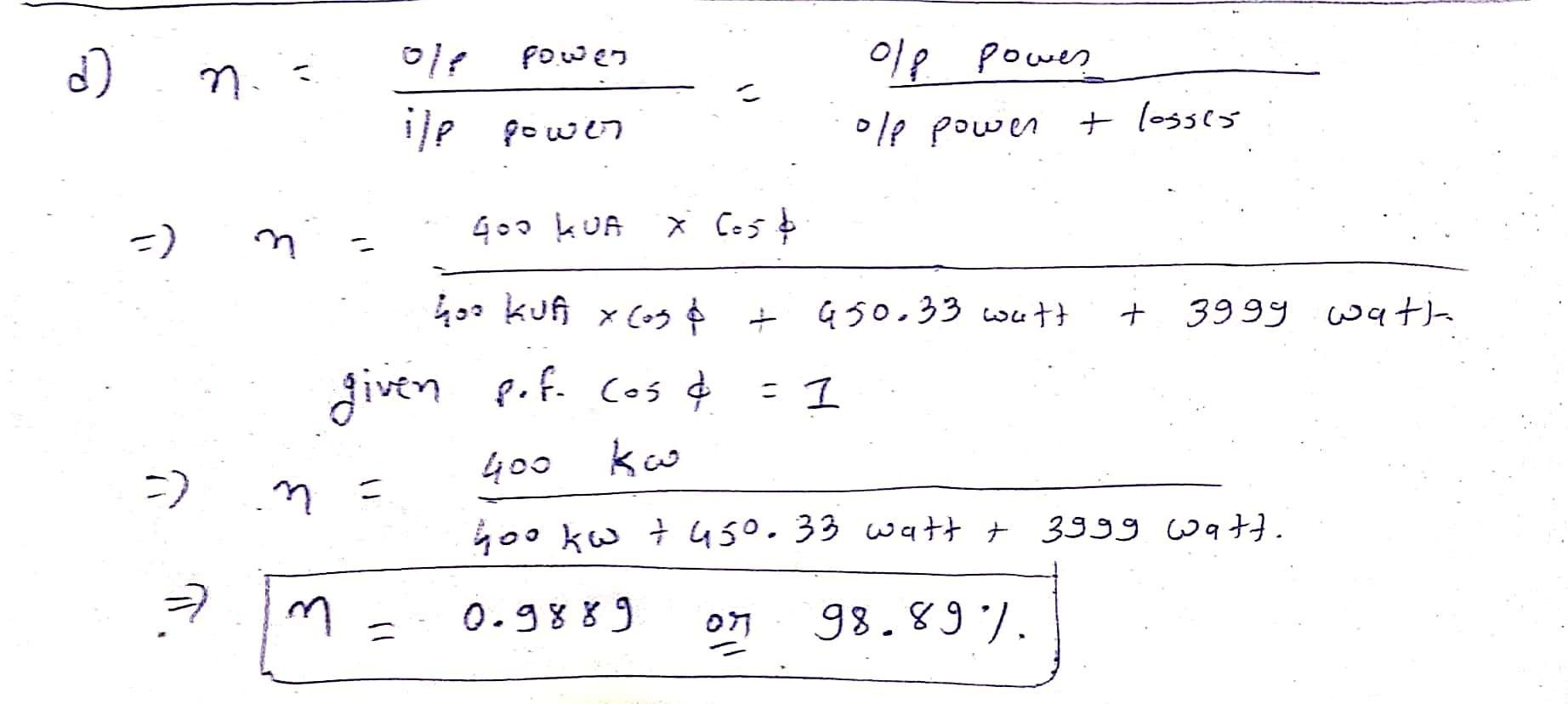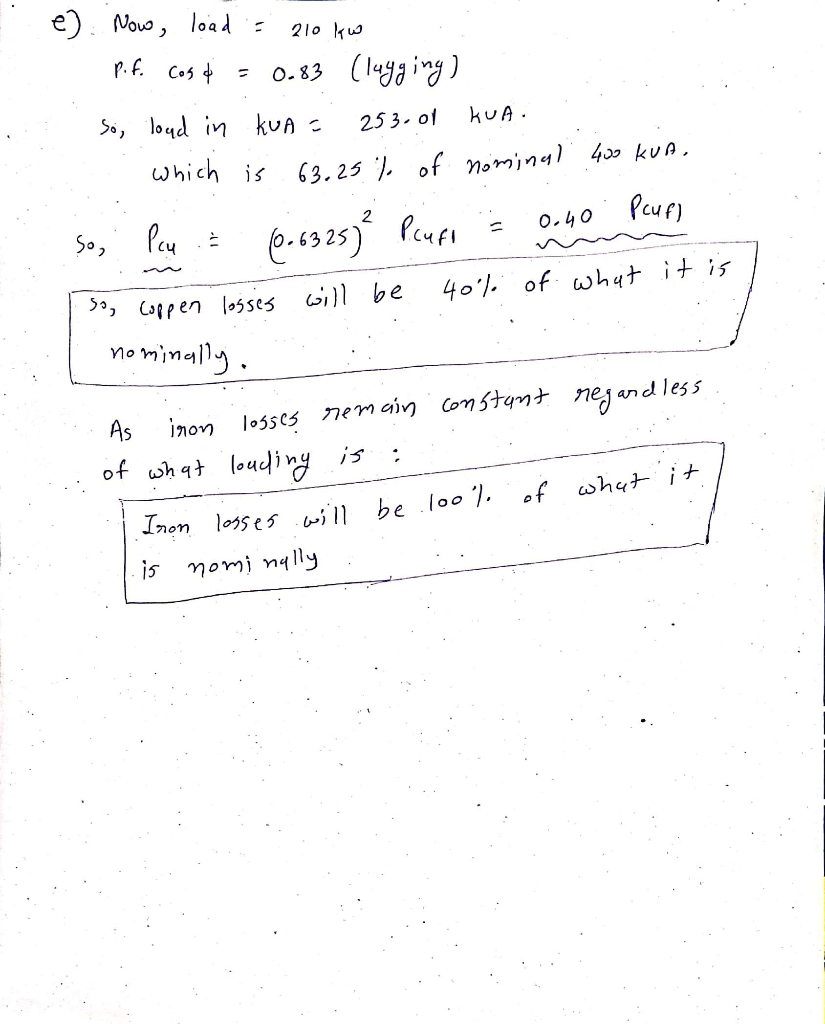##### Question

In: Electrical Engineering

# A three phase transformer of 400kVA, 10kV/400V, Dy11 is connected to its nominal load with cosφ...

A three phase transformer of 400kVA, 10kV/400V, Dy11 is connected to its nominal load with cosφ = 1.

a) Calculate the nominal primary and secondary line current.
b) Calculate the corresponding currents in the primary and secondary winding.

At an open circuit test, the primary line current is 0,52A with cosφ = 0,05 at nominal line voltage.
The short circuit test (at nominal current) shows 214V primary line voltage and P=1,333kW per phase.

c) Calculate the total iron losses and total copper losses of this transformer.

d) Calculate the efficiency η of this transformer.

Now the transformer will be loaded with 210kW with cosφ = 0,83 (lagging).

e) Give both the iron losses and the copper losses as a percentage of what they are nominally.

## Solutions

##### Expert Solution## Related Solutions

##### A balanced three-phase delta-connected load is connected to a balanced three-phase delta-connected source via a transmission...
A balanced three-phase delta-connected load is connected to a balanced three-phase delta-connected source via a transmission line. The line-line voltage for the source is 100∟0° V/phase and the impedance of load is (27 + j18) Ω/phase. The transmission line has an impedance of (1 + j4) Ω/line. a) Draw the complete schematic of the power system showing the location of wattmeters. (Two-Wattmeter system is considered for this problem). Phase “a” could be considered as a reference phase. b) What must...
##### b) The load connected to a three-phase supply comprises three similar coils connected in star. If...
b) The load connected to a three-phase supply comprises three similar coils connected in star. If the phase currents are 10A and the total active power and power factor absorb by the loads are 3kW and 0.8 lagging, respectively. Find: i. The magnitude of phase and line voltages ii. Total apparent power (1 mark) iii. The impedance of each coil. c) If the coil in Q3(b) are now connected in delta to the same three-phase supply, calculate the magnitude of...
##### Three balanced 3-phase loads are connected in parallel. Load 1 is Y-connected with an impedance of...
Three balanced 3-phase loads are connected in parallel. Load 1 is Y-connected with an impedance of 400 + j300 ? per phase, load 2 is ?-connected with an impedance of 2400 – j1800 ? per phase, and load 3 is absorbing 172.8 + j2203.2 kVA. The loads are fed from a set of distribution lines with an impedance of 2 + j16 ? per line. The magnitude of the line-to-neutral voltage at the load end of the line is 24?3...
##### A three phase transformer of 200MVA, 33kV/380kV, Yd11 is delivering nominal power at cosφ = 1....
A three phase transformer of 200MVA, 33kV/380kV, Yd11 is delivering nominal power at cosφ = 1. Calculate the nominal primary and secondary line current. Calculate the winding ratio n of this transformer. The primary line current is 15,9A during an open circuit test. The measured power per phase is then 53kW. During a short circuit test (at nominal current), 271V primary line voltage is measured at cosφ = 0,225. Calculate the total iron losses and total copper losses of this...
##### A balanced three-phase generator delivers 6.3 kW to a delta-connected load with impedance 13 - j23.4...
A balanced three-phase generator delivers 6.3 kW to a delta-connected load with impedance 13 - j23.4 Ω per phase. The magnitude of the line current ILis:
##### A three phase 10kW, 1700rpm, 60Hz, four poles, Y connected induction motor has a no-load speed...
A three phase 10kW, 1700rpm, 60Hz, four poles, Y connected induction motor has a no-load speed of 1750rpm. The rotational mechanical losses are constant and equal to 500W. The core losses are constant and equal to 300W. The copper losses in the stator equal to the copper losses in the rotor at full load. If the rotor resistance per phase is 0.25?, calculate the following: a.The frequency of the induced current in the rotor circuit at full load. b.The percentage...
##### A balanced three-phase delta-connected source has a line veltage of 120 V. It is connected to...
A balanced three-phase delta-connected source has a line veltage of 120 V. It is connected to a load via a three-wire transmission line. The per-phase impedance of the delta-connected load is 30 + 120j (2. The impedance of each wire of the transmission line is 2 + 4j 2. How much power is dissipated by the load? By the transmission line? What is the power factor of the load? What is the overall power factor?
##### Plate values of a three-phase transformer are given below: 1600 kVA, 15 kV / 0,4 kV,...
Plate values of a three-phase transformer are given below: 1600 kVA, 15 kV / 0,4 kV, 50 Hz, i0 = 1.2%; ukN= 6%, idle losses 2450 W, rated power copper losses 14500 W; Dy5 connected.. III) If there is 75% load in the power factor with 0.85 inductance and 0.85 capacity. At what times of the day can capacity loading be seen? What solution should be applied to prevent voltage change? IV) Draw the fastening group. How can this transformer...
##### Plate values of a three-phase transformer are given below: 1600 kVA, 15 kV / 0,4 kV,...
Plate values of a three-phase transformer are given below: 1600 kVA, 15 kV / 0,4 kV, 50 Hz, i0 = 1.2%; ukN= 6%, idle losses 2450 W, rated power copper losses 14500 W; Dy5 connected.. Calculate the secondary VOLTAGE separately at 75% load in the power factor with 0.85 inductance and 0.85 capacity. Compare and interpret the results. At what times of the day can capacity loading be seen? What solution should be applied to prevent voltage change?
##### A company plans to purchase a transformer that will be used 12 hours at full load...
A company plans to purchase a transformer that will be used 12 hours at full load and 12 hours with no load but still connected to the power supply at all times. The transformer has copper losses of 5 kW and core losses of 3.5 kW. Electricity costs \$0.07/kW-hr. What is the total cost of transformer losses over a period of 1 year?